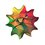RMO doubt

Find all primes $p$ and $q$ such that ${ p }^{ 2 }+7pq+{ q }^{ 2 }$ is a perfect square.Note by Swapnil Das
4 years, 2 months ago

This discussion board is a place to discuss our Daily Challenges and the math and science related to those challenges. Explanations are more than just a solution — they should explain the steps and thinking strategies that you used to obtain the solution. Comments should further the discussion of math and science.

When posting on Brilliant:

• Use the emojis to react to an explanation, whether you're congratulating a job well done , or just really confused .
• Ask specific questions about the challenge or the steps in somebody's explanation. Well-posed questions can add a lot to the discussion, but posting "I don't understand!" doesn't help anyone.
• Try to contribute something new to the discussion, whether it is an extension, generalization or other idea related to the challenge.
• Stay on topic — we're all here to learn more about math and science, not to hear about your favorite get-rich-quick scheme or current world events.

MarkdownAppears as
*italics* or _italics_ italics
**bold** or __bold__ bold

- bulleted
- list

• bulleted
• list

1. numbered
2. list

1. numbered
2. list
Note: you must add a full line of space before and after lists for them to show up correctly
paragraph 1

paragraph 2

paragraph 1

paragraph 2

> This is a quote
This is a quote
# I indented these lines
# 4 spaces, and now they show
# up as a code block.

print "hello world"
# I indented these lines
# 4 spaces, and now they show
# up as a code block.

print "hello world"
MathAppears as
Remember to wrap math in $$...$$ or $...$ to ensure proper formatting.
2 \times 3 $2 \times 3$
2^{34} $2^{34}$
a_{i-1} $a_{i-1}$
\frac{2}{3} $\frac{2}{3}$
\sqrt{2} $\sqrt{2}$
\sum_{i=1}^3 $\sum_{i=1}^3$
\sin \theta $\sin \theta$
\boxed{123} $\boxed{123}$

Sort by:

set $p^2+7pq+q^2=m^2$, so

$(p+q)^2+5pq=m^2$. So $5pq= (m+p+q)(m-p-q)$. It follows, given that $m-p-q, that

$m-p-q$ is either $5, p, q, 5p, or 5q$. We can assume $q>p$ and exclude the last possibility.

• If $m-p-q=5$, we get $m+p+q= pq$ and

$m+p+q=2p+2q+5$. So $pq=2p+2q+5$ and $(p-2)(q-2)=9$. So the only solution is p=3 and q=11 so m=19.

• If $m-p-q= p$, then $m+p+q=5q$. Then $m= 2p+q=4q-p$, which implies $p=q$. This is excluded.

• Same for $m-p-q=q$.

• If m-p-q=5p. Then m+p+q = q which is impossible.

So (3,11) and (11,3) are the only solutions;

- 4 years, 2 months ago

Did you mean p=/=q?

- 4 years, 2 months ago